# Class 9 RD Sharma Solutions – Chapter 16 Circles- Exercise 16.2

• Last Updated : 19 Oct, 2021

### Question 1. The radius of a circle is 8 cm and the length of one of its chords is 12 cm. Find the distance of the chord from the centre.

Solution: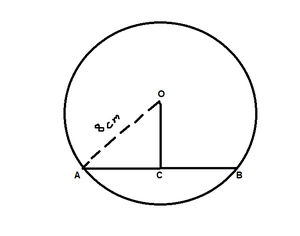Hey! Looking for some great resources suitable for young ones? You've come to the right place. Check out our self-paced courses designed for students of grades I-XII

Start with topics like Python, HTML, ML, and learn to make some games and apps all with the help of our expertly designed content! So students worry no more, because GeeksforGeeks School is now here!

According to the question, given that

Chord (AB) = 12cm

Find: the distance of the chord from the centre, i.e., OC

So, draw a perpendicular OC on AB.

As we know that, perpendicular from centre to chord bisects the chord

So, AC = BC = 12/2 = 6 cm

Now in ΔOCA,

By using Pythagoras theorem,

OA2 = AC2 + OC2

64 = 36 + OC2

OC2 = 64 – 36 = 28

OC = √28

OC = 5.291 (approx.)

Hence, the distance of the chord from the centre is 5.291 cm.

### Question 2. Find the length of a chord which is at a distance of 5 cm from the centre of a circle of radius 10 cm.

Solution: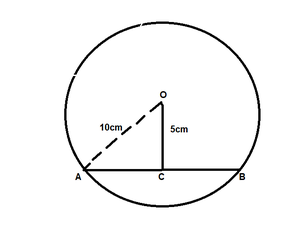According to the question, given that

Distance of the chord from the centre(OC) = 5 cm

Find: the length of a chord AB

So, In ΔOCA,

By using Pythagoras theorem,

OA2 = AC2 + OC2

100 = AC2 + 25

AC2 = 100 – 25 = 75

AC = √75 = 8.66

As we know that, perpendicular from centre to chord bisects the chord

So, AC = BC = 8.66 cm

=> AB = AC + BC = 8.66 + 8.66 = 17.32

Hence, the length of chord AB is 17.32 cm

### Question 3. Find the length of a chord which is at a distance of 4 cm from the centre of a circle of radius 6 cm.

Solution: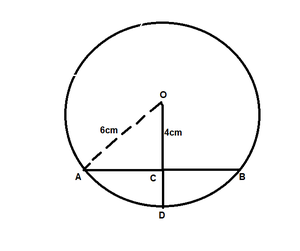According to the question, given that

Distance of the chord from the centre(OC) = 4 cm

Find: the length of a chord, i.e., AB

So, in ΔOCA,

By using Pythagoras theorem,

OA2 = AC2 + OC2

36 = AC2 + 16

AC2 = 36 – 16 = 20

AC = √20 = 4.47

AC = 4.47cm

As we know that, perpendicular from centre to chord bisects the chord

So, AC = BC = 4.47 cm

=> AB = AC + BC = 4.47 + 4.47 = 8.94

Hence, the length of chord AB is 8.94 cm

### Question 4. Two chords AB, CD of lengths 5 cm, 11 cm respectively of a circle are parallel. If the distance between AB and CD is 3 cm, find the radius of the circle.

Solution: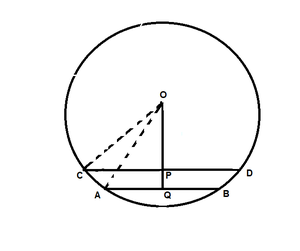According to the question, given that

The length of chord AB = 5 cm

The length of chord CD = 11 cm

PQ = 3 cm

Find: the radius of the circle, i.e., r

So, draw perpendiculars OP on CD and OQ on AB

Let us assume OP = x cm and OC = OA = r cm

As we know that, perpendicular from centre to chord bisects the chord

OP⊥CD,

So, CP = PD = 11/2 cm

And OQ⊥AB

So, AQ = BQ = 5/2 cm

Now, in ΔOCP,

By using Pythagoras theorem,

OC2 = OP2 + CP2

r2 = x2 + (11/2) 2 …..(1)

In ΔOQA,

By using Pythagoras theorem,

OA2 = OQ2 + AQ2

r2 = (x + 3)2 + (5/2)2 …..(2)

From equations (1) and (2), we get

(x + 3)2 + (5/2)2 = x2 + (11/2)2

x2 + 6x + 9 + 25/4 = x2 + 121/4

By using identity, (a + b)2 = a2 + b2 + 2ab

6x = 121/4 – 25/4 − 9

6x = 15

or x = 15/6 = 5/2

Now, substitute the value of x in equation (1), we get

r2 = (5/2)2 + (11/2) 2

r2 = 25/4 + 121/4

r2 = 146/4

r = √146/4 cm

Hence, the radius of the circle is √146/4 cm

### Question 5. Give a method to find the centre of a given circle.

Solution: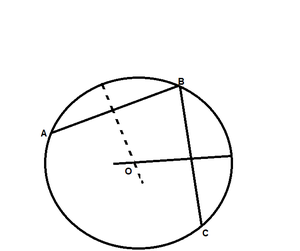Steps of Construction:

Step 1: Let us assume three points, i.e., A, B and C on a circle.

Step 2: Join AB and BC.

Step 3: Now draw perpendicular bisectors of chord AB and BC that intersect each other at a point O.

Step 4: As we know that the perpendicular bisectors of chord always pass through the centre, so the centre of the circle is point O.

### Question 6. Prove that the line joining the mid-point of a chord to the centre of the circle passes through the mid-point of the corresponding minor arc.

Solution: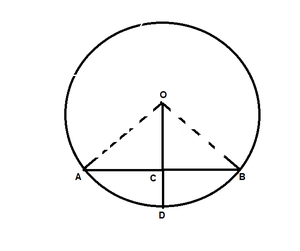According to the question,

Prove: D is the mid-point of arc AB.

Proof:

From the given figure,

Let us assume C is the mid-point of chord AB.

Now, In ΔOAC and ΔOBC

OA = OB [Radius of circle]

OC = OC [Common]

AC = BC [C is the mid-point of chord AB]

By SSS condition

ΔOAC ≅ ΔOBC

Hence, BY C.P.C.T

∠AOC = ∠BOC

Hence Proved.

### Question 7. Prove that the diameter of a circle which bisects a chord of the circle also bisects the angle subtended by the chord at the centre of the circle.

Solution: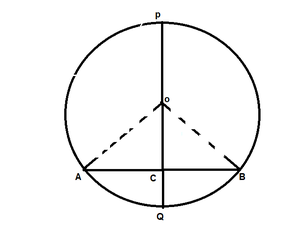According to the question,

Prove: PQ bisects ∠AOB

Proof:

Form the given figure, we get

PQ is a diameter of circle which bisects the chord AB at C.

In ΔBOC and ΔAOC

OC = OC [Common side]

AC = BC [Given]

By SSS condition

ΔAOC ≅ ΔBOC

Hence, BY C.P.C.T

∠AOC = ∠BOC

So, PQ bisects ∠AOB

Hence proved.

### Question 8. Prove that two different circles cannot intersect each other at more than two points.

Solution:

Prove: two different circles cannot intersect each other at more than two points.

Proof:

Let us considered the two circles intersect in three points, i.e., A, B and C.

Now as we know that, points A, B, and C are non-collinear.

So, a unique circle passes through these three points(i.e., A, B, C).

Now, this is a contradiction to the fact that the two given circles are passing through A, B, C.

Hence, two circles cannot intersect each other at more than two points.

Hence, proved.

### Question 9. A line segment AB is of length 5cm. Draw a circle of radius 4 cm passing through A and B. Can you draw a circle of radius 2 cm passing through A and B? Give reason in support of your answer.

Solution:

According to the question, given that

A line segment AB = 5 cm,

One circle having radius r1 = 4 cm which is passing through point A and B

and other circle having radius r2 = 2 cm.

As we know that the largest chord of any circle is equal to the diameter of that circle.

So, 2 × r2 < AB

Hence, there is no possibility to draw a circle whose diameter is smaller than the length of the chord.

### Question 10. An equilateral triangle of side 9 cm is inscribed in a circle. Find the radius of the circle.

Solution: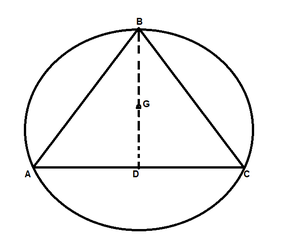According to the question,

Let us considered ABD is the equilateral triangle and the side of the triangle is 9cm

and AD is one of its median.

Find: the radius of the circle

So,

Let us assume G be centroid of ΔABC

Then AG:GD = 2:1

As we know that in an equilateral triangle, the centroid coincides with circumcenter,

So, G is centre of circumference with circumradius GA.

Also, G is the centre and GD ⊥ BC,

Now we find the radius(AG) of the circle

= 3√3 cm

Hence, the radius of the circle is 3√3 cm

### Question 11. Given an arc of circle, complete the circle.

Solution: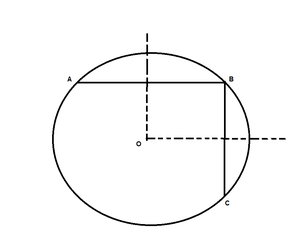Steps of Construction:

Step 1: Let us considered three points i.e., A, B and C on the given arc

Step 2: Join AB and BC

Step 3: Now draw the perpendicular bisectors of chords AB and BC which intersect each other at point O.

So, the centre of the circle is O.

Step 4: Now join OA

Step 5: Hence, the centre of the circle is O, so the radius of the circle is OA.

Hence, the complete the circle.

### Question 12. Draw different pairs of circles. How many points does each pair have in common? What is the maximum number of common points?

Solution: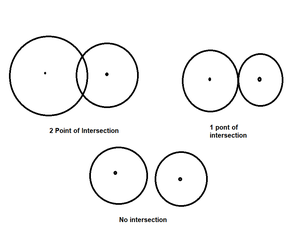Here, the first set of circles contains 2 common points, Second set of circles contains 1 common points, and

third set of circles contains 0 common points.

Hence, the maximum number of common points are 2.

### Question 13. Suppose You are given a circle. Give a construction to find its centre.

Solution:Steps Of Construction:

Step 1: Let us considered three points i.e., A, B and C on the given circle.

Step 2: Join AB And BC.

Step 3: Now draw the perpendicular bisectors of chord AB and BC which intersect each other at point O.

Step 4: As we know that the perpendicular bisectors of chord always pass through the centre, so the centre of the circle is point O.

### Question 14. Two chords AB and CD of lengths 5cm and 11cm respectively of a circle are parallel to each other and are the opposite side of its centre. If the distance between AB and CD is 6cm, find the radius of the circle.

Solution: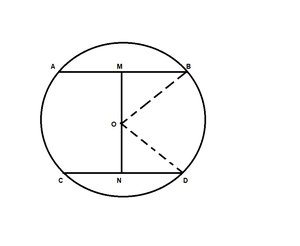According to the question, given that

The length of chod AB = 5cm

The length of chod CD = 11cm

The distance between AB and CD(i.e., MN) = 6cm

Find: the radius of the circle

So, draw OM ⊥ AB and ON ⊥ CD

Now, join OB and OD

As we know that, perpendicular from centre to chord bisects the chord

So, BM = AB/2 = 5/2

ND = CD/2 = 11/2

Let us assume ON be x, so OM will be 6 – x.

In ΔMOB,

OM2 + MB2 = OB2

(6 – x)2 + (5/2)2 = OB2

36 + x2 – 12x + 25/4 = OB2  ………(i)

In ΔNOD,

ON2 + ND2 = OD2

x2 + (11/2)2 = OD2

x2 + 121/4 = OD2  ………(ii)

We have OB = OD (radii of same circle)

So from eq(i) and (ii), we get

36 + x2 – 12x +25/4 = x2 +121/4

12x = 36 + 25/4 – 121/4

12x = 48/4

x = 1

Now put the value of x in eq(i), we get

OD2 = 1 + 121/4

OD = 5√5/2

Hence, the radius of circle is 5√5/2

### Question 15. The lengths of two parallel chords of a circle are 6cm and 8cm. If the smaller chord is at a distance of 4cm from the centre, what is the distance of the other chord from the centre?

Solution: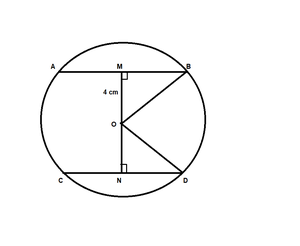According to the question, given that

The length of chod AB = 6cm

The length of chod CD = 8cm

The distance between the chord AB and the centre(OM) = 4 cm

Find: The distance between the chord CD and the centre(ON)

So, MB = AB/2 = 6/2 = 3cm

In ΔOMB,

OM2 + MB2 = OB2

42 + 92 = OB2

OB = 5cm

In ΔOND,

OD = OB = 5cm [radii of same circle]

ND = CD/2 = 8/2 = 4cm

ON2 + ND2 = OD2

ON2 + 42 = 52

ON2 = 25 – 16

ON = 3cm

Hence, The distance between the chord CD and the centre(ON) is 3cm.

My Personal Notes arrow_drop_up